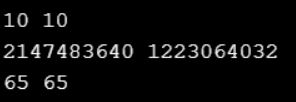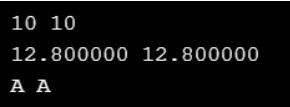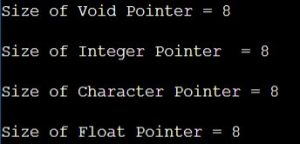# Void Pointer in C

## Void Pointer in C Language with Examples

In this article, I will discuss Void Pointer in C Language with Examples. Please read our previous articles discussing the Null Pointer in C Language with Examples.

##### Void Pointer in C Language:

A void pointer in C, also known as a generic pointer, is a type of pointer that can point to an object of any data type. It is a flexible tool, especially useful in scenarios that require a function to handle different data types or when dealing with raw memory. The Size of the void pointer is 2 bytes. When accessing the data, using a void pointer is mandatory to use the Typecasting mechanism. else it will give you an error.

###### Key Points of Void Pointers in C Langauge:
• Generic Type: A void pointer can point to a variable of any type. For example, it can point to an int, a float, a char, or even a complex data structure.
• No Dereferencing: A void pointer cannot be dereferenced directly. This is because the size of the pointed-to object is unknown. To dereference a void pointer, you must first cast it to another pointer type.
• Arithmetic Limitations: Pointer arithmetic is not allowed with void pointers in standard C, as the size of the object it points to is unknown. So, with the Void pointer, incrementation and decrementation of the pointer are restricted.
##### Program to understand Void Pointer in C Language:

Let us understand the need for a void pointer with an example. In the below program, we have created three different pointers, i.e., integer, character, and float pointers. So, in the example below, we are not using the void pointer, so we created three-pointers.

```#include<stdio.h>
void main ()
{
int i;
float f;
char ch;
int *iptr = (int *) 0;
float *fptr = (float *) 0;
char *cptr = (char *) 0;
iptr = &i;
i = 10;
printf ("\n %d %d ", i, *iptr);
fptr = &f;
f = 12.8;
printf ("\n %d %d", f, *fptr);
cptr = &ch;
ch = 'A';
printf ("\n %d %d", ch, *cptr);
}
```
###### Output:Let us see how we can replace the previous program with the void pointer. That means, instead of constructing 3 types of pointers, we can create a single pointer variable that can access and manipulate any kind of variable properly, i.e., void pointer. As the void pointer can access and manipulate any kind of data variables, it is also called a Generic Pointer.

##### Program using Void Pointer:
```#include<stdio.h>
void main ()
{
int i;
float f;
char ch;
void *ptr;
ptr = &i;
*(int *) ptr = 10;
printf ("\n %d %d", i, *(int *) ptr);
ptr = &f;
*(float *) ptr = 12.8;
printf ("\n %f %f", f, *(float *) ptr);
ptr = &ch;
*(char *) ptr = 'A';
printf ("\n %c %c", ch, *(char *) ptr);
}
```
###### Output:Note: A Void Pointer in C Programming Language is a pointer with no associated data type, which is why a void pointer in C programming language can hold the address of any data type and be typecasted to any data type.

##### Size of the Void Pointer:

The size of the void pointer in the C programming language is the same as that of the character datatype pointer. According to the C language standard, the representation of a pointer to void is the same as the pointer of a character datatype. The pointer size will vary depending on the platform we are using. For a better understanding, please have a look at the below example.

```#include <stdio.h>
int main ()
{
void *VP = NULL;		//Void Pointer
int *IP = NULL;		//Integer Pointer
char *CP = NULL;		//Character Pointer
float *FP = NULL;		//Float Pointer

printf ("Size of Void Pointer = %d\n\n", sizeof (VP));
printf ("Size of Integer Pointer  = %d\n\n", sizeof (IP));
printf ("Size of Character Pointer = %d\n\n", sizeof (CP));
printf ("Size of Float Pointer = %d\n\n", sizeof (FP));

return 0;
}```
###### Output:##### Example: Basic Usage of Void Pointers
```#include <stdio.h>

int main() {
int a = 10;
float b = 5.5;
void *ptr;

ptr = &a; // ptr points to an int
printf("Value of a: %d\n", *(int *)ptr);

ptr = &b; // now ptr points to a float
printf("Value of b: %f\n", *(float *)ptr);

return 0;
}```

In this example, ptr is a void pointer pointing to an int and then a float. Notice the casting of ptr to the appropriate type before dereferencing.

##### Example: Void Pointers with Functions

Void pointers are particularly useful in functions that work with different data types.

```#include <stdio.h>

void printValue(void *ptr, char type) {
switch (type) {
case 'i':
printf("Integer: %d\n", *(int *)ptr);
break;
case 'f':
printf("Float: %f\n", *(float *)ptr);
break;
}
}

int main() {
int a = 10;
float b = 5.5;

printValue(&a, 'i');
printValue(&b, 'f');

return 0;
}```

Here, printValue is a generic function that can handle different data types based on the type argument.

##### Example: Void Pointers in Dynamic Memory Allocation

Dynamic memory allocation functions (malloc, calloc, and realloc) return a void pointer. This allows them to be used to allocate memory for any data type.

```#include <stdio.h>
#include <stdlib.h>

int main() {
int *ptr = malloc(5 * sizeof(int)); // Allocating memory for 5 integers

if (ptr == NULL) {
printf("Memory allocation failed.\n");
return 1;
}

// Use the allocated memory
for (int i = 0; i < 5; i++) {
ptr[i] = i * 10;
printf("%d ", ptr[i]);
}

free(ptr);

return 0;
}
```

In this example, malloc returns a void pointer, which is then cast to an int *.

##### Example: Using Void Pointers in Data Structures

Void pointers can be used in data structures for storing generic data.

```#include <stdio.h>

typedef struct {
void *data;
// Other fields...
} GenericNode;

int main() {
int data = 42;
GenericNode node;

node.data = &data;

// Cast back to int pointer to retrieve the value
printf("Node data: %d\n", *((int *)node.data));

return 0;
}
```
##### Key Points:
• Void pointers can work with different data types using a single pointer.
• They are essential in creating generic functions and are widely used in dynamic memory allocation.
• Casting is necessary for dereferencing and performing operations with void pointers.
• While void pointers increase the flexibility of code, they should be used with caution to ensure type safety and to avoid runtime errors.
##### When to Use Void Pointer in C Language?

Here are some common scenarios where void pointers are particularly useful:

• Dynamic Memory Allocation: Functions like malloc(), calloc(), and realloc() deal with void pointers (void*). This allows these functions to allocate memory without knowing the type of data that will be stored in that memory.
• Generic Data Structures: When implementing data structures like linked lists, trees, queues, or hash tables that are meant to store different types of data, void pointers can be used to hold data of any type.
• Generic Functions: When you want a function to accept data of any type, you can use a void pointer as the parameter to create generic functions. This is common in functions for libraries or APIs where the functions should be general-purpose and not specific to a data type.
• Interfacing with System Calls or Libraries: Some system calls or library functions use void pointers to deal with different data types generically. For example, the qsort() function in the C standard library uses void pointers to sort arrays of any data type.
• Callback Functions: When writing libraries or frameworks where you don’t know what data the callback functions will need to handle, void pointers can be used to pass data to callbacks.
##### Important Considerations
• Type Safety: Since void pointers do not know what type of data they point to, it’s easy to accidentally misuse them, leading to bugs or undefined behavior. Always ensure that void pointers are correctly cast to the appropriate type before use.
• Pointer Arithmetic: Arithmetic operations are not allowed on void pointers directly, as their size is unknown. You must cast them to another pointer type if you need to perform pointer arithmetic.
• Dereferencing: Void pointers cannot be dereferenced directly. They must be cast to another pointer type before you can dereference them.